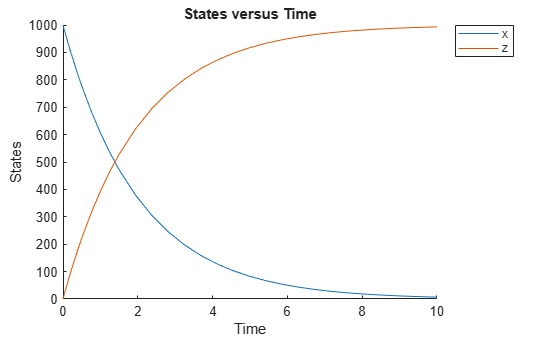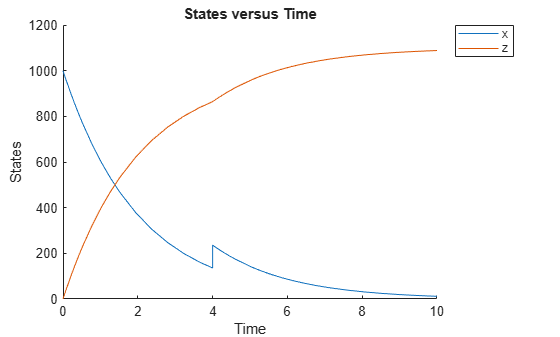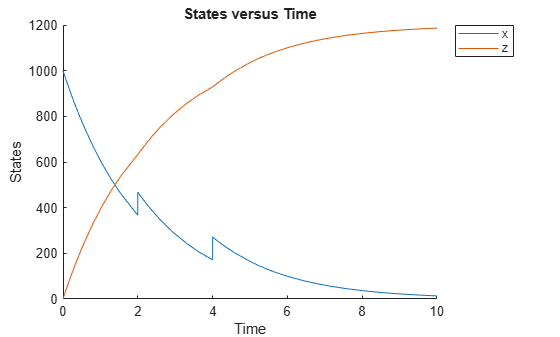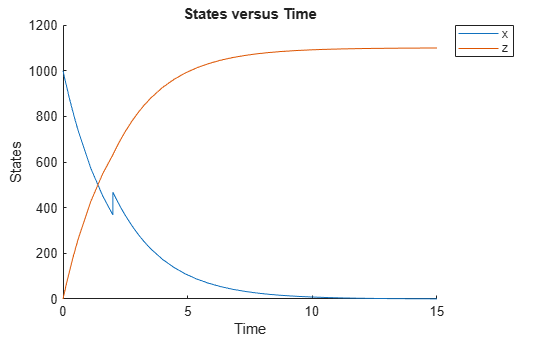# sbiosimulate

Simulate SimBiology model

## Syntax

``````[time,x,names] = sbiosimulate(modelObj)``````
``````[time,x,names] = sbiosimulate(modelObj,csObj)``````
``````[time,x,names] = sbiosimulate(modelObj,dvObj)``````
``````[time,x,names] = sbiosimulate(modelObj,csObj,dvObj)``````
``````[time,x,names] = sbiosimulate(modelObj,csObj,variantObj,doseObj)``````
``simDataObj = sbiosimulate(___)``

## Description

example

``````[time,x,names] = sbiosimulate(modelObj)``` returns simulation results in three outputs, `time`, vector of time samples, `x`, simulation data, and `names`, column labels of simulation data `x`. This function simulates the SimBiology® model `modelObj` while using the active configuration set along with its active doses and active variants if any.```

example

``````[time,x,names] = sbiosimulate(modelObj,csObj)``` returns simulation results using the specified configset object `csObj`, any active variants, and any active doses. Any other configsets are ignored. If you set `csObj` to empty `[]`, the function uses the active configset.```

example

``````[time,x,names] = sbiosimulate(modelObj,dvObj)``` returns simulation results using doses or variants specified by `dvObj` and the active configset. `dvObj` can be one of the following: `Variant object` `ScheduleDose object` `RepeatDose object` Array of doses or variants If you set `dvObj` to empty `[]`, the function uses the active configset, active variants, and active doses.If you specify `dvObj` as variants, the function uses the specified variants and active doses. Any other variants are ignored.If you specify `dvObj` as doses, the function uses the specified doses and active variants. Any other doses are ignored.```

example

``````[time,x,names] = sbiosimulate(modelObj,csObj,dvObj)``` returns simulation results using a configset object `csObj` and dose, variant, or an array of doses or variants specified by `dvObj`. If you set `csObj` to `[]`, then the function uses the active configset object. If you set `dvObj` to `[]`, then the function uses no variants, but uses active doses.If you specify `dvObj` as variants, the function uses the specified variants and active doses. Any other variants are ignored.If you specify `dvObj` as doses, the function uses the specified doses and active variants. Any other doses are ignored.```

example

``````[time,x,names] = sbiosimulate(modelObj,csObj,variantObj,doseObj)``` returns simulation results using a configset object `csObj`, variant object or variant array specified by `variantObj`, and dose object or dose array specified by `doseObj`. If you set `csObj` to `[]`, then the function uses the active configset object. If you set `variantObj` to `[]`, then the function uses no variants. If you set `doseObj` to `[]`, then the function uses no doses.```

example

````simDataObj = sbiosimulate(___)` returns simulation results in a `SimData object` `simDataObj` using any of the input arguments in the preceding syntaxes.```

## Examples

collapse all

`sbioloadproject radiodecay.sbproj`

Change the simulation stop time to 15 seconds.

```csObj = getconfigset(m1,'active'); set(csObj,'Stoptime',15);```

Simulate the model and return outputs in an array.

`[t,x,n] = sbiosimulate(m1);`

Plot the simulated results for species x and z.

```figure; plot(t,x) xlabel('Time') ylabel('States') title('States vs Time') legend('species x','species z')```You can also return the results to a SimData object.

`simData = sbiosimulate(m1);`

Plot the simulated results.

`sbioplot(simData);``sbioloadproject radiodecay.sbproj`

Add two doses of 100 molecules each for species x, scheduled at 2 and 4 seconds respectively.

```dObj1 = adddose(m1,'d1','schedule'); dObj1.Amount = 100; dObj1.AmountUnits = 'molecule'; dObj1.TimeUnits = 'second'; dObj1.Time = 2; dObj1.TargetName = 'unnamed.x'; dObj2 = adddose(m1,'d2','schedule'); dObj2.Amount = 100; dObj2.AmountUnits = 'molecule'; dObj2.TimeUnits = 'second'; dObj2.Time = 4; dObj2.TargetName = 'unnamed.x';```

Simulate the model using no dose or any subset of the dose array.

```sim1 = sbiosimulate(m1); sim2 = sbiosimulate(m1,dObj1); sim3 = sbiosimulate(m1,dObj2); sim4 = sbiosimulate(m1,[dObj1,dObj2]);```

Plot the results.

`sbioplot(sim1);``sbioplot(sim2);``sbioplot(sim3);``sbioplot(sim4);``sbioloadproject radiodecay.sbproj`

Create a new configset with a stop time of 15.

```cs1 = addconfigset(m1,'cs1'); cs1.StopTime = 15;```

Add a scheduled dose of 100 molecules at 2 seconds for species x.

```dObj = adddose(m1,'d1','schedule'); dObj.Amount = 100; dObj.AmountUnits = 'molecule'; dObj.TimeUnits = 'second'; dObj.Time = 2; dObj.TargetName = 'unnamed.x';```

Simulate the model using configset and dose objects.

`sim = sbiosimulate(m1,cs1,dObj);`

Plot the result.

`sbioplot(sim);``sbioloadproject radiodecay.sbproj`

Add a new configuration set using a stop time of 15 seconds.

```csObj = m1.addconfigset('newStopTimeConfigSet'); csObj.StopTime = 15;```

Add a scheduled dose of 100 molecules at 2 seconds for species x.

```dObj = adddose(m1,'d1','schedule'); dObj.Amount = 100; dObj.AmountUnits = 'molecule'; dObj.TimeUnits = 'second'; dObj.Time = 2; dObj.TargetName = 'unnamed.x';```

Add a variant of species x using a different initial amount of 500 molecules.

```vObj = addvariant(m1,'v1'); addcontent(vObj,{'species','x','InitialAmount',500});```

Simulate the model using the same configset, variant, and dose objects. Use the same order of input arguments as shown next.

`sim = sbiosimulate(m1,csObj,vObj,dObj);`

Plot the result.

`sbioplot(sim);`## Input Arguments

collapse all

SimBiology model, specified as a SimBiology model object. The model minimally needs one reaction or rate rule for simulations.

Configuration set object, specified as a `configset object` that stores simulation-specific information. When you specify `csObj` as `[]`, `sbiosimulate` uses the currently active configset object.

If your model contains events, the `csObj` object cannot specify `'expltau'` or `'impltau'` for the `SolverType` property.

If your model contains doses, the `csObj` object cannot specify `'ssa'`, `'expltau'`, or `'impltau'` for the `SolverType` property.

Dose or variant object, specified as a `ScheduleDose object` , `RepeatDose object` , an array of dose objects, `Variant object` , or an array of variant objects.

• Use `[]` when you want to explicitly exclude any variant objects from the `sbiosimulate` function.

• When `dvObj` is a dose object, `sbiosimulate` uses the specified dose object as well as any active variant objects if available.

• When `dvObj` is a variant object, `sbiosimulate` uses the specified variant object as well as any active dose objects if available.

Variant object, specified as a `Variant object` or an array of variant objects. Use `[]` when you want to explicitly exclude any variant objects from `sbiosimulate`.

Dose object, specified as a ```ScheduleDose object``` , `RepeatDose object` , or an array of dose objects. A dose object defines additions that are made to species amounts or parameter values. Use `[]` when you want to explicitly exclude any dose objects from `sbiosimulate`.

## Output Arguments

collapse all

Vector of time samples, returned as an `n-by-1` vector containing the simulation time steps. `n` is the number of time samples.

Simulation data, returned as an `n-by-m` data array, where `n` is the number of time samples and `m` is the number of states logged in the simulation. Each column of `x` describes the variation in the quantity of a species, compartment, or parameter over time.

Names of species, compartments, or parameters, returned as an `m-by-1` cell array of character vectors. In other words, `names` contains the column labels of the simulation data, `x`. If the species are in multiple compartments, species names are qualified with the compartment name in the form `compartmentName.speciesName`.

Simulation data, returned as a ```SimData object``` that holds time and state data as well as metadata, such as the types and names for the logged states or the configuration set used during simulation. You can access time, data, and names stored in a `SimData object` by using its properties.

## Version History

Introduced before R2006a